# Central Science: Chapter 5 Flashcards

Set Details Share
created 3 years ago by Chemistry4Life
3,647 views
updated 3 years ago by Chemistry4Life
College: First year, College: Second year, College: Third year, College: Fourth year
Subjects:
chemistry
Page to share:
Embed this setcancel
COPY
code changes based on your size selection
Size:
X

1

Objects can possess energy as ________.

(a) endothermic energy
(b) potential energy
(c) kinetic energy

A) a only
B) b only
C) c only
D) a and c
E) b and c

E

2

The internal energy of a system is always increased by ________.
A) adding heat to the system
B) having the system do work on the surroundings
C) withdrawing heat from the system
D) adding heat to the system and having the system do work on the surroundings
E) a volume decompression

A

3

The internal energy of a system ________.
A) is the sum of the kinetic energy of all of its components
B) is the sum of the rotational, vibrational, and translational energies of all of its components
C) refers only to the energies of the nuclei of the atoms of the component molecules
D) is the sum of the potential and kinetic energies of the components
E) none of the above

D

4

Which one of the following conditions would always result in an increase in the internal energy of a system?
A) The system loses heat and does work on the surroundings.
B) The system gains heat and does work on the surroundings.
C) The system loses heat and has work done on it by the surroundings.
D) The system gains heat and has work done on it by the surroundings.
E) None of the above is correct.

D

5

When a system ________, ΔE is always negative.
A) absorbs heat and does work
B) gives off heat and does work
C) absorbs heat and has work done on it
D) gives off heat and has work done on it
E) None of the above is always negative.

B

6

Which one of the following is an endothermic process?
A) ice melting
B) water freezing
C) boiling soup
D) Hydrochloric acid and barium hydroxide are mixed at 25 °C: the temperature increases.
E) Both A and C

E

7

Which one of the following is an exothermic process?
A) ice melting
B) water evaporating
C) boiling soup
D) condensation of water vapor
E) Ammonium thiocyanate and barium hydroxide are mixed at 25 °C: the temperature drops.

D

8

Of the following, which one is a state function?
A) H
B) q
C) w
D) heat
E) none of the above

A

9

Which of the following is a statement of the first law of thermodynamics?
A) Ek = 1/2mv2
B) A negative ΔH corresponds to an exothermic process.
C) ΔE = Efinal - Einitial
D) Energy lost by the system must be gained by the surroundings.
E) 1 cal = 4.184 J (exactly)

D

10

The internal energy can be increased by ________.

(a) transferring heat from the surroundings to the system
(b) transferring heat from the system to the surroundings
(c) doing work on the system

A) a only
B) b only
C) c only
D) a and c
E) b and c

D

11

A ________ ΔH corresponds to an ________ process.
A) negative, endothermic
B) negative, exothermic
C) positive, exothermic
D) zero, exothermic
E) zero, endothermic

B

12

A ________ ΔH corresponds to an ________ process.
A) negative, endothermic
B) positive, exothermic
C) positive, endothermic
D) zero, exothermic
E) zero, endothermic

C

13

ΔH for an endothermic process is ________ while ΔH for an exothermic process is ________.
A) zero, positive
B) zero, negative
C) positive, zero
D) negative, positive
E) positive, negative

E

14

For a given process at constant pressure, w is positive. This means that the process involves ________.
A) work being done by the system on the surroundings
B) work being done by the surroundings on the system
C) no work being done
D) an equal amount of work done on the system and by the system
E) work being done against a vacuum

A

15

Which one of the following statements is true?
A) Enthalpy is an intensive property.
B) The enthalpy change for a reaction is independent of the state of the reactants and products.
C) Enthalpy is a state function.
D) H is the value of q measured under conditions of constant volume.
E) The enthalpy change of a reaction is the reciprocal of the ΔH of the reverse reaction.

C

16

All of the following statements are true except ________.
A) Internal energy is a state function.
B) The enthalpy change for a reaction is equal in magnitude, but opposite in sign, to the enthalpy change for the reverse reaction.
C) The enthalpy change for a reaction depends on the state of the reactants and products.
D) The enthalpy of a reaction is equal to the heat of the reaction.
E) Enthalpy is an intensive property.

E

17

A chemical reaction that absorbs heat from the surroundings is said to be ________ and has a ________ ΔH at constant pressure.
A) endothermic, positive
B) endothermic, negative
C) exothermic, negative
D) exothermic, positive
E) exothermic, neutral

A

18

A chemical reaction that releases heat to the surroundings is said to be ________ and has a ________ ΔH at constant pressure.
A) endothermic, positive
B) endothermic, negative
C) exothermic, negative
D) exothermic, positive
E) exothermic, neutral

C

19

The reaction

4Al (s) + 3O2 (g) → 2 Al2O3 (s) ΔH° = -3351 kJ
is ________, and therefore heat is ________ by the reaction.
A) endothermic, released
B) endothermic, absorbed
C) exothermic, released
D) exothermic, absorbed
E) thermoneutral, neither released nor absorbed

C

20

When ________ is constant, the enthalpy change of a process equal to the amount of heat transferred into or out of the system?
A) temperature
B) volume
C) pressure and volume
D) temperature and volume
E) pressure

E

21

The units of heat capacity are ________.
A) K/J or °C/J
B) J/K or J/°C
C) J/g-K or J/g-°C
D) J/mol
E) g-K/J or g-°C/J

B

22

The units of specific heat are ________.
A) K/J or °C/J
B) J/K or J/°C
C) J/g-K or J/g-°C
D) J/mol
E) g-K/J or g-°C/J

C

23

The term Btu which stands for ________ is commonly used in engineering applications.
A) Best thermal unit
B) Bunsen thermal unit
C) British thermal unit
D) Bake thermal unit
E) Brush thermal unit

C

24

Which of the following is a statement of Hess's law?
A) If a reaction is carried out in a series of steps, the ΔH for the reaction will equal the sum of the enthalpy changes for the individual steps.
B) If a reaction is carried out in a series of steps, the ΔH for the reaction will equal the product of the enthalpy changes for the individual steps.
C) The ΔH for a process in the forward direction is equal in magnitude and opposite in sign to the ΔH for the process in the reverse direction.
D) The ΔH for a process in the forward direction is equal to the ΔH for the process in the reverse direction.
E) The ΔH of a reaction depends on the physical states of the reactants and products.

A

25

For which one of the following reactions is ΔH°rxn equal to the heat of formation of the product?
A) N2 (g) + 3H2 (g) → 2NH3 (g)
B) (1/2)N2 (g) + O2 (g) → NO2(g)
C) 6C (s) + 6H (g) → C6H6 (l)
D) P (g) + 4H (g) + Br (g) → PH4Br (l)
E) 12C (g) + 11H2 (g) + 11O (g) → C6H22O11 (g)

B

26

Of the following, ΔH°f is not zero for ________.
A) O2 (g)
B) C (graphite)
C) N2 (g)
D) F2 (s)
E) Cl2 (g)

D

27

Of the following, ΔH°f is not zero for ________.
A) Sc (g)
B) Si (s)
C) P4 (s, white)
D) Br2 (l)
E) Ca (s)

A

28

Consider the following two reactions:

A → 2B ΔH°rxn = 456.7 kJ/mol
A → C ΔH°rxn = -22.1 kJ/mol

Determine the enthalpy change for the process:

2B → C
A) -478.8 kJ/mol
B) -434.6 kJ/mol
C) 434.6 kJ/mol
D) 478.8 kJ/mol

A

29

In the reaction below, ΔH°f is zero for ________.
Ni (s) + 2CO (g) + 2PF3 (g) → Ni(CO)2(PF3)2 (l)

A) Ni (s)
B) CO (g)
C) PF3 (g)
D) Ni(CO)2(PF3)2 (l)
E) both CO (g) and PF3 (g)

A

30

For the combustion reaction of methane, ΔH°f is zero for ________.
CH4 (g) + O2 (g) → 2H2O(g) + CO2 (g)

A) O2 (g)
B) CH4 (g)
C) CO2 (g)
D) H2O (g)
E) Both O2 (g) and CH4 (g)

A

31

For the following reactions, the ΔH°rxn is NOT equal to ΔH°f for the product except for ________.
A) CH4 (g) + 2Cl2 (g) → CH2Cl2 (l) + 2HCl (g)
B) N2 (g) + O3 (g) → N2O3 (g)
C) Xe (g) + 2F2 (g) → XeF4 (g)
D) 2CO (g) + O2 (g) → 2CO2 (g)
E) C (diamond) + O2 (g) → CO2 (g)

C

32

For the following reactions, the ΔH°rxn is NOT equal to ΔH°f for the product except for ________.
A) 2Ca (s) + O2 (g) → 2CaO (s)
B) 3Mg (s) + N2 (g) → Mg3N2 (s)
C) C2H2 (g) + H2 (g) → H4 (g)
D) 2C (graphite) + O2 (g) → 2CO (g)
E) C (diamond) + O2 (g) → CO2 (g)

B

33

For the following reactions, the ΔH°rxn is NOT equal to ΔH°f for the product except for ________.
A) N2 (g) + O2 (g) → 2NO (g)
B) 2H2 (g) + O2 (g) → 2H2O (l)
C) 2H2 (g) + O2 (g) → 2H2O (g)
D) 2C (s, graphite) + 2H2(g) → C2H4 (g)
E) H2O (l) + 1/2 O2 (g) → H2O2 (l)

D

34

For the following reactions, the ΔH°rxn is NOT equal to ΔH°f for the product except for ________.
A) H2O (l) + 1/2O2 (g) → H2O2 (l)
B) N2 (g) + O2 (g) → 2NO (g)
C) 2H2 (g) + O2 (g) → 2H2O (l)
D) 2H2 (g) + O2 (g) → 2H2O (g)
E) none of the above

E

35

For the following reactions, the ΔH°rxn is NOT equal to ΔH°f for the product except for ________.
A) H2 (g) + 1/2 O2 (g) → H2O (l)
B) H2 (g) + O2 (g) → H2O2 (l)
C) 2C (s, graphite) + 2H2 (g) → C2H4 (g)
D) 1/2 N2 (g) + O2 (g) → NO2 (g)
E) all of the above

E

36

The term standard conditions with respect to enthalpy change means ________.
A) 1 atm and 0 K
B) 1 atm and 1 L
C) 1 atm and 298 K
D) 1 L and 0 K
E) 1 atm and 1 °C

C

37

The energy released by combustion of ________ of a substance is called the fuel value of the substance.
A) 1 kJ
B) 1 kg
C) 1 lb
D) 1 J
E) 1 g

E

38

Fuel values of hydrocarbons increase as the ________ increases.
A) C atomic ratio
B) H/C atomic ratio
C) H atomic ratio
D) C/C atomic ratio
E) C/H atomic ratio

B

39

________ yields the highest fuel value.
A) hydrogen
B) charcoal
C) bituminous coal
D) natural gas
E) wood

A

40

All of the following are considered fossil fuels except ________.
A) hydrogen
B) anthracite coal
C) crude oil
D) natural gas
E) petroleum

A

41

The most abundant fossil fuel is ________.
A) natural gas
B) petroleum
C) coal
D) uranium
E) hydrogen

C

42

Calculate the kinetic energy in J of an electron moving at 6.00 × 106 m/s. The mass of an electron is 9.11 × 10-28 g.
A) 4.98 × 10-48 J
B) 3.28 × 10-14 J
C) 1.64 × 10-17 J
D) 2.49 × 10-48 J
E) 6.56 × 10-14 J

C

43

Calculate the kinetic energy in joules of an automobile weighing 2135 lb and traveling at 55 mph.
A) 1.2 × 104 J
B) 2.9 × 105 J
C) 5.9 × 105 J
D) 3.2 × 106 J
E) 3.2 × 10-6 J

B

44

Calculate the kinetic energy in joules of an automobile weighing 4345 lb and traveling at 75 mph.
A) 5.5 × 105 J
B) 5.5 × 10-5 J
C) 1.1 × 106 J
D) 2.2 × 106 J
E) 2.2 × 10-6 J

C

45

The kinetic energy of a 7.3 kg steel ball traveling at 18.0 m/s is ________ J.
A) 1.2 × 103
B) 66
C) 2.4 × 103
D) 1.3 × 102
E) 7.3

A

46

The kinetic energy of a 10.3 g golf ball traveling at 48.0 m/s is ________ J.
A) 1.20 × 103
B) 66
C) 11.9
D) 1.3 × 102
E) 23.7

C

47

Calculate the kinetic energy in joules of a 150 lb jogger (68.1 kg) traveling at 12.0 mile/hr (5.36 m/s).
A) 1.96 × 103 J
B) 365 J
C) 978 J
D) 183 J
E) 68.1 J

C

48

What is the kinetic energy of a 55.2 g object moving at 135 m/s.
A) 503 J
B) 5.03 × 105 J
C) 1.01 × 103 J
D) 3.73 J
E) 3.73 × 103 J

A

49

The kinetic energy of a 23.2-g object moving at a speed of 81.9 km/hr is ________ J.
A) 1900
B) 77.8
C) 145
D) 1.43 × 10-3
E) 6.00

E

50

What is the kinetic energy of a 145 g baseball traveling at 89.9 mi/hr?
A) 1.17 × 105 J
B) 2.91 J
C) 234 J
D) 5.83
E) 117 J

E

51

A 100-watt electric incandescent light bulb consumes ________ J of energy in 24 hours. [1 Watt (W) = 1 J/sec]
A) 2.40 × 103
B) 8.64 × 103
C) 4.17
D) 2.10 × 103
E) 8.64 × 106

E

52

The ΔE of a system that releases 12.4 J of heat and does 4.2 J of work on the surroundings is ________ J.
A) 16.6
B) 12.4
C) 4.2
D) -16.6
E) -8.2

D

53

The ΔE of a system that absorbs 12.4 J of heat and does 4.2 J of work on the surroundings is ________ J.
A) 16.6
B) 12.4
C) 4.2
D) -16.6
E) 8.2

E

54

The change in the internal energy of a system that absorbs 2,500 J of heat and that does 7,655 J of work on the surroundings is ________ J.
A) 10,155
B) 5,155
C) -5,155
D) -10,155
E) 1.91 × 107

C

55

The change in the internal energy of a system that releases 2,500 J of heat and that does 7,655 J of work on the surroundings is ________ J.
A) -10,155
B) -5,155
C) -1.91 × 107
D) 10,155
E) 5,155

A

56

Hydrogen gas and bromine gas react to form hydrogen bromide gas. How much heat (kJ) is released when 155 grams of HBr is formed in this reaction? ΔH° = -72 kJ.
A) 137
B) 69
C) -69
D) -137
E) 1.12 × 105

B

57

The value of ΔH° for the reaction below is -126 kJ. ________ kj are released when 2.00 mol of NaOH is formed in the reaction?

2Na2O2 (s) + 2H2O (l) → 4NaOH (s) + O2 (g)

A) 252
B) 63
C) 3.9
D) 7.8
E) -126

B

58

The value of ΔH° for the reaction below is -790 kJ. The enthalpy change accompanying the reaction of 0.95 g of S is ________ kJ.
2S (s) + 3O2 (g) → 2SO3 (g)

A) 23
B) -23
C) -12
D) 12
E) -790

C

59

The value of ΔH° for the reaction below is -6535 kJ. ________ kJ of heat are released in the combustion of 16.0 g of (l)?
2C6H6 (l) + 15O2 (g) → 12CO2 (g) + 6H2O (l)

A) 1.34 × 103
B) 5.23 × 104
C) 669
D) 2.68 × 103
E) -6535

C

60

Carbon monoxide and oxygen gas react to form carbon dioxide. How much heat is released when 89.5 grams of O2 (g) reacts with excess CO? ΔH° = -482 kJ.
A) 1.35 × 103 kJ
B) 2.70 × 103 kJ
C) 1.35 × 10-3 kJ
D) 674 kJ
E) 4.31 × 104 kJ

A

61

The value of ΔH° for the reaction below is -336 kJ. Calculate the heat (kJ) released to the surroundings when 23.0 g of HCl is formed.

CH4 (g) + 3Cl2 (g) → CHCl3 (l) + 3HCl (g)

A) 177 kJ
B) 2.57 × 103 kJ
C) 70.7 kJ
D) 211 kJ
E) -336 kJ

C

62

How much heat is released when 29.5 grams of Cl2 (g) reacts with excess hydrogen?

H2 (g) + Cl2 (g) → 2HCl (g) ΔH° = -186 kJ.

A) 186 kJ
B) 310 kJ
C) -77.4 kJ
D) -186 kJ
E) 77.4 kJ

E

63

The enthalpy change for the following reaction is -483.6 kJ:

2H2 (g) + O2 (g) → 2H2O (g)

Therefore, the enthalpy change for the following reaction is ________ kJ.
4H2 (g) + 2O2 (g) → 4H2O (g)
A) -483.6
B) -967.2
C) 2.34 × 105
D) 483.6
E) 967.2

B

64

The value of ΔH° for the reaction below is +128.1 kJ:

CH3OH (l) → CO (g) + 2H2 (g)
How many kJ of heat are consumed when 15.5 g of C OH (l) decomposes as shown in the equation?
A) 0.48 kJ
B) 62.0 kJ
C) 1.3 × 102 kJ
D) 32 kJ
E) 8.3 kJ

B

65

The value of ΔH° for the reaction below is +128.1 kJ:

CH3OH (l) → CO (g) + 2H2 (g)
How much heat is consumed when 87.1 g of hydrogen gas is formed?
A) 2.76 × 103 kJ
B) 5.52 × 103 kJ
C) -5.52 × 103 kJ
D) -2.76 × 103 kJ
E) -128.1 kJ

A

66

The value of ΔH° for the reaction below is +128.1 kJ:

CH3OH (l) → CO (g) + 2H2 (g)
How many kJ of heat are consumed when 5.10 g of CO (g) is formed as shown in the equation?
A) 0.182 kJ
B) 162 kJ
C) 8.31 kJ
D) 23.3 kJ
E) 62.0 kJ

D

67

CH3OH (l) decomposes into carbon monoxide and hydrogen gas in the presence of heat. How much heat is consumed when 5.75 g of CO (g) is formed? ΔH° = +128.1 kJ.
A) 26.3 kJ
B) 23.3 kJ
C) 62.0 kJ
D) 162 kJ
E) 8.3 kJ

A

68

The value of ΔH° for the reaction below is -1107 kJ:

2Ba (s) + O2 (g) → 2BaO (s)
How many kJ of heat are released when 5.75 g of Ba (s) reacts completely with oxygen to form
A) 96.3 kJ
B) 26.3 kJ
C) 46.4 kJ
D) 23.2 kJ
E) 193 kJ

D

69

The value of ΔH° for the reaction below is -1107 kJ:

2Ba (s) + O2 (g) → 2BaO (s)
How many kJ of heat are released when 5.75 g of BaO (s) is produced?
A) 56.9 kJ
B) 23.2 kJ
C) 20.8 kJ
D) 193 kJ
E) 96.3 kJ

C

70

How many kJ of heat are released when 15.75 g of Ba (s) reacts completely with oxygen gas to form BaO (s)? ΔH° = -1107 kJ.
A) 63.5 kJ
B) 20.8 kJ
C) 114 kJ
D) 70.3 kJ
E) 35.1 kJ

A

71

The molar heat capacity of an unknown substance is 92.1 J/mol-K. If the unknown has a molar mass of 118 g/mol, what is the specific heat (J/g-K) of this substance?
A) 1.28
B) -92.1
C) 1.09 × 104
D) 0.781
E) 92.1

D

72

The specific heat capacity of lead is 0.13 J/g-K. How much heat (in J) is required to raise the temperature of of lead from 22 °C to 37 °C?
A) 2.0 J
B) -0.13 J
C) 5.8 × 10-4 J
D) 29 J
E) 0.13 J

D

73

The temperature of a 15-g sample of lead metal increases from 22 °C to 37 °C upon the addition of 29.0 J of heat. The specific heat capacity of the lead is ________ J/g-K.
A) 7.8
B) 1.9
C) 29
D) 0.13
E) -29

D

74

What is the molar heat capacity (in J/mol-K) of liquid bromine? The specific heat of liquid bromine is 0.226 J/g-K.
A) 36.1 J/mol-K
B) 707 J/mol-K
C) 18.1 J/mol-K
D) 9.05 J/mol-K
E) 0.226 J/mol-K

A

75

The specific heat of liquid bromine is 0.226 J/g-K. How much heat (J) is required to raise the temperature of 10.0 mL of bromine from 25.00 °C to 27.30 °C? The density of liquid bromine: 3.12 g/mL.
A) 5.20 J
B) 16.2 J
C) 300 J
D) 32.4 J
E) 10.4 J

B

76

ΔH for the reaction

IF5 (g) → IF3 (g) + F2 (g)

is ________ kJ, given the data below.
IF (g) + F2 (g) → IF3 (g) ΔH = -390 kJ

IF (g) + 2F2 (g) → IF5 (g) ΔH = -745 kJ

A) +355
B) -1135
C) +1135
D) +35
E) -35

A

77

Given the following reactions
Fe2O3 (s) + 3CO (s) → 2Fe (s) + 3CO2 (g) ΔH = -28.0 kJ

3Fe (s) + 4CO2(s) → 4CO (g) + Fe3O4(s) ΔH = +12.5 kJ

the enthalpy of the reaction of Fe2O3 with CO
3Fe2O3 (s) + CO (g) → CO2 (g) + 2Fe3O4 (s)

is ________ kJ.
A) -59.0
B) 40.5
C) -15.5
D) -109
E) +109

A

78

Given the following reactions
N2 (g) + 2O2 (g) → 2NO2 (g) ΔH = 66.4 kJ

2NO (g) + O2 (g) → 2NO2 (g) ΔH = -114.2 kJ
the enthalpy of the reaction of the nitrogen to produce nitric oxide
N2 (g) + O2 (g) → 2NO (g)

is ________ kJ.
A) 180.6
B) -47.8
C) 47.8
D) 90.3
E) -180.6

A

79

Given the following reactions
2NO → N2 + O2 ΔH = -180 kJ

2NO + O2 → 2NO2 ΔH = -112 kJ

the enthalpy of the reaction of nitrogen with oxygen to produce nitrogen dioxide

N2 + 2O2 → 2NO2

is ________ kJ.
A) 68
B) -68
C) -292
D) 292
E) -146

A

80

Given the following reactions
2S (s) + 3O2 (g) → 2SO3 (g) ΔH = -790 kJ

S (s) + O2 (g) → SO2(g) ΔH = -297 kJ

the enthalpy of the reaction in which sulfur dioxide is oxidized to sulfur trioxide

2SO2 (g) + O2 (g) → 2SO3 (g)

is ________ kJ.
A) 196
B) -196
C) 1087
D) -1384
E) -543

B

81

Given the following reactions
CaCO3 (s) → CaO (s) + CO2 (g) ΔH = 178.1 kJ
C (s, graphite) + O2(g) → CO2(g) ΔH = -393.5 kJ
the enthalpy of the reaction
CaCO3 (s) → CaO (s) + C (s, graphite) + O2 (g)

is ________ kJ.
A) 215.4
B) 571.6
C) -215.4
D) -571.6
E) 7.01 × 104

B

82

Given the following reactions
H2O (l) → H2O (g) ΔH = 44.01 kJ
2H2 (g) + O2 (g) → 2H2O (g) ΔH = -483.64 kJ
the enthalpy for the decomposition of liquid water into gaseous hydrogen and oxygen
2H2O (l) → 2H2 (g) + O2 (g)

is ________ kJ.
A) -395.62
B) -527.65
C) 439.63
D) 571.66
E) 527.65

D

83

Given the following reactions

N2 (g) + O2 (g) → 2NO (g) ΔH = +180.7 kJ
2NO( g) + O2 (g) → 2NO2 (g) ΔH = -113.1 kJ
the enthalpy for the decomposition of nitrogen dioxide into molecular nitrogen and oxygen
2NO2 (g) → N2 (g) + 2O2 (g)

is ________ kJ.
A) 67.6
B) -67.6
C) 293.8
D) -293.8
E) 45.5

B

84

Given the following reactions
N2 (g) + O2 (g) → 2NO (g) ΔH = +180.7 kJ
2NO (g) + O2(g) → 2N (g) ΔH = -113.1 kJ
the enthalpy of reaction for
4NO (g) → 2NO2 (g) + N2 (g)

is ________ kJ.
A) 67.6
B) 45.5
C) -293.8
D) -45.5
E) 293.8

C

85

Given the following reactions
N2 (g) + O2 (g) → 2NO (g) ΔH = +180.7 kJ
2N2O (g) → O2 (g) + 2N2 (g) ΔH = -163.2 kJ
the enthalpy of reaction for
2N2O (g) → 2NO (g) + N2 (g)

is ________ kJ.
A) 145.7
B) 343.9
C) -343.9
D) 17.5
E) -145.7

D

86

The value of ΔH° for the reaction below is -186 kJ.
H2 (g) + Cl2 (g) → 2HCl (g)

The value of ΔH°f for HCl (g) is ________ kJ/mol.
A) -3.72 × 102
B) -1.27 × 102
C) -93.0
D) -186
E) +186

C

87

The value of ΔH° for the following reaction is -3351 kJ:
2Al (s) + 3O2(g) → 2Al2O3(s)
The value of ΔH°f for Al2 (s) is ________ kJ.
A) -3351
B) -1676
C) -32.86
D) -16.43
E) +3351

B

88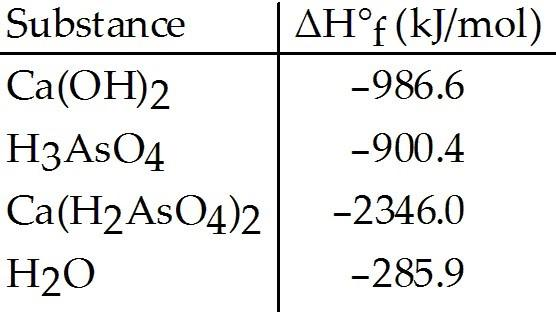Given the data in the table, ΔH°rxn for the reaction

Ca(OH)2 + 2H3AsO4 → Ca(H2AsO4)2 + 2H2O

is ________ kJ.

A) -744.9
B) -4519
C) -4219
D) -130.4
E) -76.4

D

89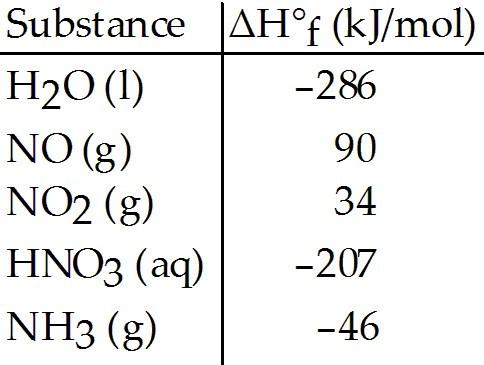Given the data in the table, ΔH°rxn for the reaction

4NH3 (g) + 5O2 (g) → 4NO (g) + 6H2O (l)

is ________ kJ.

A) -1172
B) -150
C) -1540
D) -1892
E) The ΔH°f of O2 (g) is needed for the calculation.

A

90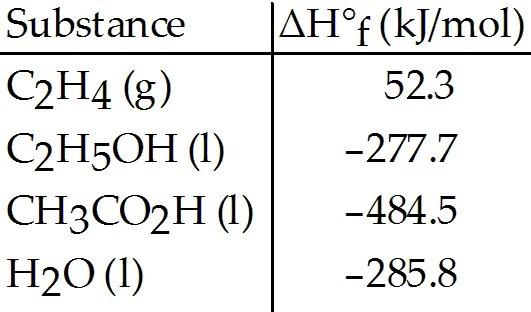Given the data in the table, ΔH°rxn for the reaction
C2H5OH (l) + O2 (g) → CH3CO2H (l) + H2O (l)

is ________ kJ.

A) -79.0
B) -1048.0
C) -476.4
D) -492.6
E) The value of ΔH°f of O2 (g) is required for the calculation.

D

91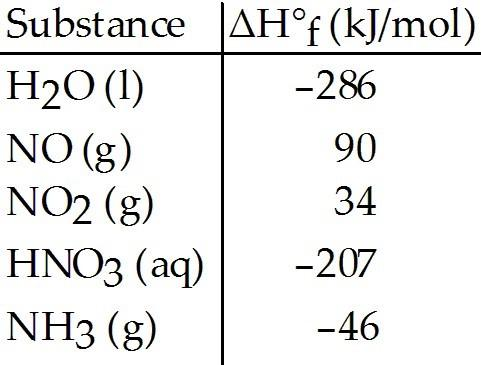Given the data in the table, ΔH°rxn for the reaction
3NO2 (g) + H2O (l) → 2HNO3 (aq) + NO (g)

is ________ kJ.

A) 64
B) 140
C) -140
D) -508
E) -64

C

92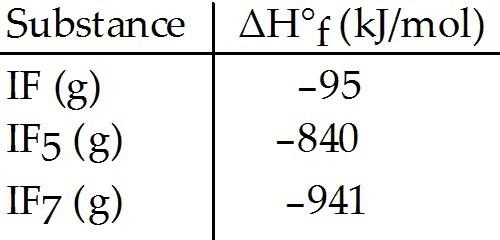Given the data in the table, ΔH°rxn for the reaction

IF5 (g) + F2 (g) → IF7 (g)

is ________ kJ.

A) 1801
B) -1801
C) 121
D) -121
E) -101

E

93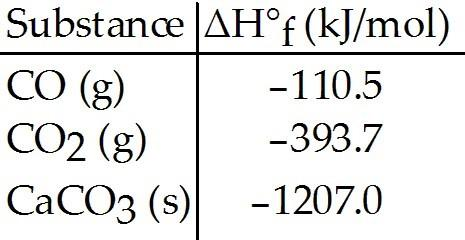Given the data in the table, ΔH° for the reaction
2CO (g) + O2 (g) → 2CO2 (g)

is ________ kJ.

A) -566.4
B) -283.2
C) 283.2
D) -677.0
E) The ΔH°f of O2 (g) is needed for the calculation.

A

94The value of ΔH° for the following reaction is 177.8 kJ. The value of Δ for CaO(s) is ________ kJ/mol.
CaCO3 (s) → CaO (s) + CO2 (g)

A) -1600
B) -813.4
C) -635.5
D) 813.4
E) 177.8

C

95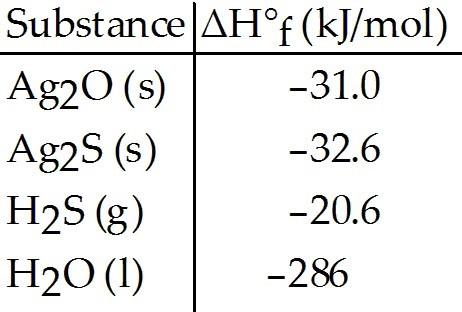Given the data in the table, ΔH°rxn for the reaction
2Ag2S (s) + O2 (g) → 2Ag2O (s) + 2S (s)

is ________ kJ.

A) -1.6
B) +1.6
C) -3.2
D) +3.2
E) The ΔH°f of S (s) and of O2 (g) are needed for the calculation.

D

96Given the data in the table, ΔH°rxn for the reaction
Ag2O (s) + H2S (g) → Ag2S (s) + H2O (l)

is ________ kJ.

A) -267
B) -370
C) -202
D) -308
E) More data are needed to complete the calculation.

A

97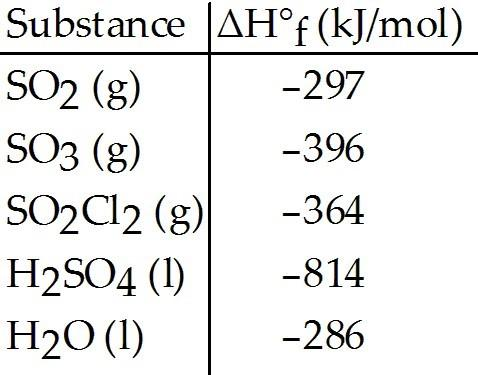Given the data in the table, ΔH°rxn for the reaction
2SO2 (g) + O2 (g) → 2SO3 (g)

is ________ kJ.

A) -99
B) 99
C) -198
D) 198
E) The ΔH°f of O2 (g) is needed for the calculation.

C

98Given the data in the table, ΔH°rxn for the reaction
SO3 (g) + H2O (l) → H2SO4 (l)

is ________ kJ.

A) -132
B) 1496
C) 704
D) -704
E) -2.16 × 103

A

99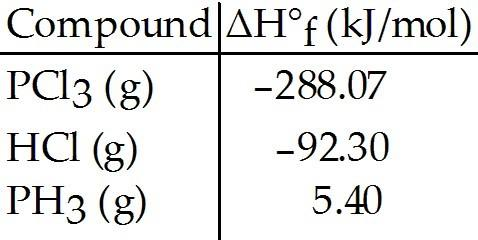Given the data in the table, ΔH°rxn for the reaction
3Cl2 (g) + PH3 (g) → PCl3 (g) + 3HCl (g)

is ________ kJ.

A) -385.77
B) -570.37
C) 570.37
D) 385.77
E) The ΔH°f of Cl2 (g) is needed for the calculation.

B

100Given the data in the table, ΔH°rxn for the reaction
PCl3 (g) + 3HCl (g) → 3Cl2 (g) + PH3 (g)

is ________ kJ.

A) -570.37
B) -385.77
C) 570.37
D) 385.77
E) The ΔH°f of Cl2 (g) is needed for the calculation.

C

101Given the data in the table and ΔH°rxn for the reaction
SO2Cl2 (g) + 2H2O (l) → H2SO4 (l) + 2HCl (g) ΔH° = -62 kJ

ΔH°f of HCl (g) is ________ kJ/mol.

A) -184
B) 60
C) -92
D) 30
E) Insufficient data are given.

C

102

A 19.5 g candy bar contains 8% protein, 33% fat, and 18% carbohydrate. The respective fuel values for protein, fat, and carbohydrate are 17, 38, and 17 kJ/g, respectively. What is the fuel value (kJ) for this piece of candy?
A) 241
B) 331
C) 27
D) 60.0
E) 17.0

B

103

A 3.00 L pitcher of sweetened ice tea contains 600. g of sugar. Assuming that the sugar is the only fuel source, what is the fuel value (in kJ) of a 250. mL serving? The respective fuel values for protein, fat, and carbohydrate are 17, 38, and 17 kJ/g, respectively.
A) 8.50 × 102 kJ
B) 10.2 × 104 kJ
C) 2.55 × 103 kJ
D) 38 kJ
E) 17 kJ

A

104

A typical fast food meal consists of a burger, fries, and a soft-drink and contains 58.0 grams of fat, 39.0 grams of protein, and 177 grams of carbohydrate. If jogging burns 950.0 kJ/hour, how many minutes would it take to completely burn off the meal? The respective fuel values for protein, fat, and carbohydrate are 17, 38, and 17 kJ/g, respectively.
A) 6.19
B) 208
C) 371
D) 17.3
E) 9.70

C

105

A slice of cake contains 29.0 grams of fat, 9.0 grams of protein, and 77 grams of carbohydrate. If swimming burns 1000.0 kJ/hour, how many minutes would it take to completely burn off the slice of cake? The respective fuel values for protein, fat, and carbohydrate are 17, 38, and 17 kJ/g, respectively.
A) 154
B) 2.56
C) 23.4
D) 117
E) 262

A

106

A 26.9 g rock rolls down the hill at a speed of 81.9 m/s . What is the kinetic energy of the rock?
A) 90.2 J
B) 145 J
C) 0.950 J
D) 90200 J
E) 1450 J

A

107

A 23.2 g piece of space debris is traveling at 81.9 m/s. What is the kinetic energy of the space debris?
A) 145 J
B) 1450 J
C) 0.950 J
D) 77800 J
E) 77.8 J

E

108

At what velocity (m/s) must a object be moving in order to possess a kinetic energy of 1.0J?
A) 0.35 m/s
B) 2.8 m/s
C) 0.13 m/s
D) 0.031 m/s
E) 0.016 m/s

A

109

At what velocity (m/s) must a 417.3 g object be moving in order to possess a kinetic energy of 3.2J?
A) 0.12 m/s
B) 26 m/s
C) 0.015 m/s
D) 0.0038 m/s
E) 0.00024 m/s

A

110

When work is done on a system, w will be a ________ value.
A) positive
B) negative
C) very large
D) very small
E) There is not enough information given to determine the answer.

A

111

The value of ΔE for a system that performs 139 kJ of work on its surroundings and gains of heat is ________ kJ.
A) -85
B) 193
C) 7506
D) 85
E) -193

A

112

The value of ΔE for a system that performs 19 kJ of work on its surroundings and loses of heat is ________ kJ.
A) -28
B) 28
C) 171
D) 10
E) -10

A

113

Calculate the work (kJ) done during a reaction in which the internal volume expands from to against an outside pressure of 2.5atm.
A) -7.3 kJ
B) 17 kJ
C) 7.3 kJ
D) -17 kJ
E) 0 kJ; No work is done.

A

114

Calculate the work (kJ) done during a reaction in which the internal volume expands from to against a vacuum (an outside pressure of 0 atm).
A) 0; kJ No work is done.
B) 3.6 kJ
C) -3.6 kJ
D) 6.5 kJ
E) -6.5 kJ

A

115

Calculate the work (kJ) done during a reaction in which the internal volume contracts from to against an outside pressure of 4.4 atm.
A) 31 kJ
B) 43 kJ
C) -31 kJ
D) -43 kJ
E) 0 kJ; No work is done.

A

116

The value of ΔE for a system that performs 151 kJ of work on its surroundings and loses 79 kJ of heat is ________ kJ.
A) +230.
B) -230.
C) +72
D) -72
E) -151

B

117

Calculate the value of ΔE in joules for a system that loses 115 J of heat and has 150 J of work performed on it by the surroundings.
A) -115 J
B) -35 J
C) +35 J
D) +265 J
E) -265 J

C

118

The value of ΔH° for the reaction below is -72 kJ. ________ kJ of heat are released when 5.5 mol of HBr is formed in this reaction.

H2 (g) + Br2 (g) → 2HBr (g)

A) 144
B) 72
C) 0.44
D) 198
E) -72

D

119

The value of ΔH° for the reaction below is -126 kJ. The amount of heat that is released by the reaction of 10.0 g of Na2O2 with water is ________ kJ.

2Na2O2 (s) + 2H2O (l) → 4NaOH (s) + O2 (g)

A) 8.08
B) 16.2
C) 67.5
D) 32.3
E) -126

A

120

The value of ΔH° for the reaction below is -482 kJ. Calculate the heat (kJ) released to the surroundings when 10.0 g of CO (g) reacts completely.
2CO (g) + O2 (g) → 2CO2 (g)

A) 2410 kJ
B) 172 kJ
C) 86.0 kJ
D) 482 kJ
E) -482 kJ

C

121

In the presence of excess oxygen, methane gas burns in a constant-pressure system to yield carbon dioxide and water:

CH4 (g) + 2O2 (g) → CO2 (g) + 2H2O (l) △H = -890.0 kJ

Calculate the value of q (kJ) in this exothermic reaction when 1.80 g of methane is combusted at constant pressure.
A) -100.1 kJ
B) 0.0324 kJ
C) -0.0100 kJ
D) 30.9 kJ
E) -1.00 × 105 kJ

A

122

Hydrogen peroxide decomposes to water and oxygen at constant pressure (△H = -196 kJ). What is the value of q (kJ) for this reaction when 4.60 g of hydrogen peroxide decomposes at constant pressure?
A) -26.5 kJ
B) -0.0189 kJ
C) 1.25 kJ
D) -2.65 × 104 kJ
E) -13.3 kJ

E

123

The combustion of titanium with oxygen produces titanium dioxide:

Ti (s) + O2(g) → TiO2 (s)

When 0.610 g of titanium is combusted in a bomb calorimeter, the temperature of the calorimeter increases from 25.00 °C to 50.50 °C. In a separate experiment, the heat capacity of the calorimeter is measured to be 9.84 kJ/K. The heat of reaction for the combustion of a mole of Ti in this calorimeter is ________ kJ/mol.
A) 2.09
B) 4.14
C) -311
D) -0.154
E) -1.98 × 104

E

124

A sample of aluminum metal absorbs 11.2 J of heat, upon which the temperature of the sample increases from 23.2 °C to 30.5 °C. Since the specific heat capacity of aluminum is 0.90 J/g-K, the mass of the sample is ________ g.
A) 72
B) 1.7
C) 10.
D) 65
E) 7.3

B

125

A sample of calcium carbonate [CaCO3 (s)] absorbs of heat, upon which the temperature of the sample increases from 20.8 °C to 27.3 °C. If the specific heat of calcium carbonate is what is the mass (in grams) of the sample?
A) 7.6 g
B) 5.1 g
C) -7.6 g
D) 0.13 g
E) 5.3 g

A

126

How many joules of heat are absorbed when the temperature of a 13.9 g sample of CaCO3 (s) increases from 21.7 °C to 33.3 °C? Specific heat of calcium carbonate is 0.82 J/g-K.
A) 130 J
B) 0.68 J
C) s-130 J
D) -0.68 J
E) 9.5 J

A

127

An 6.11 g sample of calcium carbonate [CaCO3 (s)] absorbs of heat, upon which the temperature of the sample increases from 19.2 °C to 35.9 °C. What is the specific heat of calcium carbonate?
A) 0.82 J/g-K
B) -0.82 J/g-K
C) 31 J/g-K
D) 230 J/g-K
E) 8600 J/g-K

A

128

A sample of iron absorbs 81.0 J of heat, upon which the temperature of the sample increases from 19.7 °C to 28.2 °C. If the specific heat of iron is 0.450 J/g-K, what is the mass (in grams) of the sample?
A) 21.2 g
B) 4.29 g
C) -21.2 g
D) 0.0472 g
E) 3.83 g

A

129

The temperature of a 35.1 g sample of iron increases from 24.6 °C to 31.8 °C If the specific heat of iron is 0.450 J/g-K, how many joules of heat are absorbed?
A) 115 J
B) 0.0936 J
C) -115 J
D) 0.722 J
E) 3.29 J

A

130

A 22.9 g sample of iron absorbs 155 J of heat, upon which the temperature of the sample increases from 23.9 °C to 38.9 °C. What is the specific heat of iron?
A) 0.451 J/g-K
B) -0.451 J/g-K
C) 237 J/g-K
D) 102 J/g-K
E) 53,200 J/g-K

A

131

The specific heat capacity of liquid water is 4.18 J/g-K. How many joules of heat are needed to raise the temperature of 7.25 g of water from 20.0 °C to 44.1 °C?
A) 41.8 J
B) 730 J
C) 1.94 × 103 J
D) 2.39 × 10-2 J
E) 66.8 J

B

132

The specific heat capacity of methane gas is 2.20 J/g-K. How many joules of heat are needed to raise the temperature of 7.25 g of methane from 22.0 °C to 57.0 °C?
A) 115 J
B) 558 J
C) 1.26 × 103 J
D) 8.67 × 10-3 J
E) 41.3 J

B

133

The specific heat capacity of liquid mercury is 0.14 J/g-K. How many joules of heat are needed to raise the temperature of 6.00 g of mercury from 25.1 °C to 65.3 °C?
A) 1.7 × 103 J
B) 34 J
C) 76 J
D) 5.8 × 10-4 J
E) 2.2 J

B

134

How much heat is required to raise the temperature of a 1.15 kg piece of copper metal from 25.0 °C to 77.5 °C? The specific heat capacity of solid copper metal is 0.385 J/g-K.
A) 2.32 × 104 J
B) 1.57 × 105 J
C) 23.2 J
D) 6.38 × 10-6 J
E) 0.00638 J

A

135

A 4.50-g sample of liquid water at 25.0 °C is heated by the addition of 133 J of energy. The final temperature of the water is ________ °C. The specific heat capacity of liquid water is 4.18 J/g-K.
A) 149
B) 25.1
C) -17.9
D) 32.1
E) 7.07

D

136

A 10.1 g sample of NaOH is dissolved in 250.0 g of water in a coffee-cup calorimeter. The temperature increases from 23.0 °C to ________°C. Specific heat of liquid water is 4.18 J/g-K and ΔH for the dissolution of sodium hydroxide in water is 44.4 kJ/mol.
A) 35.2
B) 24.0
C) 33.7
D) 33.3
E) 40.2

D

137

A 50.0-g sample of liquid water at 25.0 °C is mixed with 23.0 g of water at 79.0 °C. The final temperature of the water is ________ °C.
A) 123
B) 27.3
C) 52.0
D) 231
E) 42.0

E

138

A 5.00-g sample of copper metal at 25.0 °C is heated by the addition of 133 J of energy. The final temperature of the copper is ________ °C. The specific heat capacity of copper is
A) 35.1
B) 25.0
C) 45.0
D) 95.0
E) 70.0

D

139

The temperature of a 24.3 g sample of gold increases from 23.7 °C to 31.5 °C. If the specific heat of gold is 0.129 J/g-K, how many joules of heat are absorbed?
A) 24.5 J
B) 0.0414 J
C) -24.5 J
D) 0.293 J
E) 1.01 J

A

140

What is the enthalpy change (in kJ) of a chemical reaction that raises the temperature of 250.0 mL of solution having a density of 1.25 g/mL by 3.33 °C? (The specific heat of the solution is 3.74 J/g-K.)
A) -7.43 kJ
B) -12.51 kJ
C) 8.20 kJ
D) -3.89 kJ
E) 6.51 kJ

D

141

An 8 oz. bottle of energy drink contains 6.0 g of protein, 2.0 g of fat, and 16.3 g of carbohydrate. The fuel value of this energy drink bottle is ________ kJ. The fuel values for protein, fat, and carbohydrate are 17, 38, and 17 kJ/g, respectively.
A) 520
B) 280
C) 720
D) 460
E) 72

D

142

________ is defined as the energy used to move an object against a force.

Work

143

The ΔHvap of water is 40.7 kJ at 100 °C. How much liquid water in grams can be converted to vapor if 5950 J of heat are absorbed?

2.63 grams

144

The ΔHvap of water is 40.7 kJ at 100 °C. How much heat energy is required to convert 15.0 grams of liquid water to vapor.

33.9 kJ

145

When 0.800 grams of NaOH is dissolved in 100.0 grams of water, the temperature of the solution increases from 25.00 °C to 27.06 °C. The amount of heat absorbed by the water is ________ J. (The specific heat of water is 4.18 J/g-°C.)

868

146

The ΔHrxn for the combustion of methane is -890.0 kJ. How much heat energy (kJ) is released if 82.1 grams of methane are burned in an excess amount of oxygen?

4555 kJ

147

The ________ of a reaction is the enthalpy change when all reactants and products are at 1 atm pressure and a specific temperature.

standard enthalpy change

148

Coal contains hydrocarbons of high molecular weight as well as compounds containing ________, sulfur, or nitrogen.

oxygen

149

Work equals mass times distance.

false

150

One joule equals 1 kg-m2/s2.

true

151

Energy units include watts, volts, and newtons.

false

152

The primary component of natural gas is methane.

true

153

Renewable energy sources are essentially exhaustible.

false

154

Petroleum is a liquid that can be refined to produce fuels such as gasoline, diesel oil, and kerosene.

true